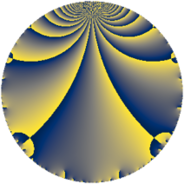# Properties

 Label 210.3.cLevel $210$ Weight $3$ Character orbit 210.c Rep. character $\chi_{210}(29,\cdot)$ Character field $\Q$ Dimension $24$ Newform subspaces $1$ Sturm bound $144$ Trace bound $0$

# Related objects

## Defining parameters

 Level: $$N$$ $$=$$ $$210 = 2 \cdot 3 \cdot 5 \cdot 7$$ Weight: $$k$$ $$=$$ $$3$$ Character orbit: $$[\chi]$$ $$=$$ 210.c (of order $$2$$ and degree $$1$$) Character conductor: $$\operatorname{cond}(\chi)$$ $$=$$ $$15$$ Character field: $$\Q$$ Newform subspaces: $$1$$ Sturm bound: $$144$$ Trace bound: $$0$$

## Dimensions

The following table gives the dimensions of various subspaces of $$M_{3}(210, [\chi])$$.

Total New Old
Modular forms 104 24 80
Cusp forms 88 24 64
Eisenstein series 16 0 16

## Trace form

 $$24 q + 48 q^{4} + 44 q^{9} + O(q^{10})$$ $$24 q + 48 q^{4} + 44 q^{9} + 16 q^{10} + 4 q^{15} + 96 q^{16} - 80 q^{19} - 28 q^{21} + 48 q^{25} - 56 q^{30} + 224 q^{31} + 128 q^{34} + 88 q^{36} - 92 q^{39} + 32 q^{40} - 72 q^{45} - 144 q^{46} - 168 q^{49} - 284 q^{51} - 144 q^{54} - 320 q^{55} + 8 q^{60} + 192 q^{64} + 224 q^{66} - 152 q^{69} - 56 q^{70} + 48 q^{75} - 160 q^{76} - 72 q^{79} - 212 q^{81} - 56 q^{84} - 64 q^{85} - 240 q^{90} + 168 q^{91} - 128 q^{94} - 876 q^{99} + O(q^{100})$$

## Decomposition of $$S_{3}^{\mathrm{new}}(210, [\chi])$$ into newform subspaces

Label Dim $A$ Field CM Traces $q$-expansion
$a_{2}$ $a_{3}$ $a_{5}$ $a_{7}$
210.3.c.a $24$ $5.722$ None $$0$$ $$0$$ $$0$$ $$0$$

## Decomposition of $$S_{3}^{\mathrm{old}}(210, [\chi])$$ into lower level spaces

$$S_{3}^{\mathrm{old}}(210, [\chi]) \cong$$ $$S_{3}^{\mathrm{new}}(15, [\chi])$$$$^{\oplus 4}$$$$\oplus$$$$S_{3}^{\mathrm{new}}(30, [\chi])$$$$^{\oplus 2}$$$$\oplus$$$$S_{3}^{\mathrm{new}}(105, [\chi])$$$$^{\oplus 2}$$